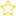See the live dashboard for CensusAtSchool 2023

This standard is derived from Achievement Objective S8.4, Investigate situations that involve elements of chance.

• calculating probabilities of independent, combined and conditional events

Students should be able to:

• Understand true probability vs model estimates vs experimental estimates
• Randomness, independence mutually exclusive events, conditional probability
• Probability distribution tables and graphs
• Two way tables, probability tress, venn diagrams

This standard requires students to understand the relationship between true probability, model estimates, and experimental estimates.

Sigma notation (PDF 224KB) may be required, for example in calculations and proofs.

• Keyword: Probability
• (Last updated: 03/05/18. Added: 03/05/18)
• ## Rate this resource:(No ratings yet)